Next: Angular Momentum Up: Wave Mechanics Previous: Three-Dimensional Wave Mechanics

# Simple Harmonic Oscillator

The classical Hamiltonian of a simple harmonic oscillator is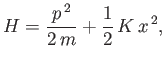(C.106)

where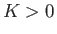is the so-called force constant of the oscillator. Assuming that the quantum-mechanical Hamiltonian has the same form as the classical Hamiltonian, the time-independent Schrödinger equation for a particle of mass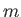and energy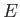moving in a simple harmonic potential becomes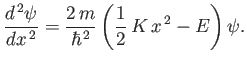(C.107)

Let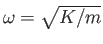, where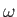is the oscillator's classical angular frequency of oscillation. Furthermore, let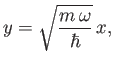(C.108)

and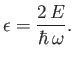(C.109)

Equation (C.107) reduces to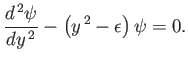(C.110)

We need to find solutions to the previous equation that are bounded at infinity. In other words, solutions that satisfy the boundary condition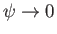as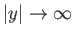.

Consider the behavior of the solution to Equation (C.110) in the limit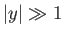. As is easily seen, in this limit, the equation simplifies somewhat to give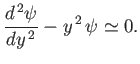(C.111)

The approximate solutions to the previous equation are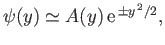(C.112)

where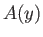is a relatively slowly-varying function of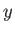. Clearly, if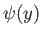is to remain bounded asthen we must chose the exponentially decaying solution. This suggests that we should write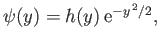(C.113)

where we would expect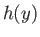to be an algebraic, rather than an exponential, function of.

Substituting Equation (C.113) into Equation (C.110), we obtain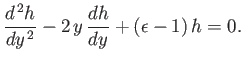(C.114)

Let us attempt a power-law solution of the form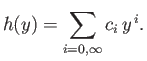(C.115)

Inserting this test solution into Equation (C.114), and equating the coefficients of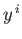, we obtain the recursion relation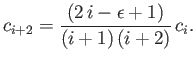(C.116)

Consider the behavior ofin the limit. The previous recursion relation simplifies to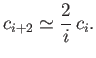(C.117)

Hence, at large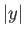, when the higher powers ofdominate, we have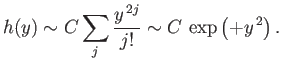(C.118)

It follows that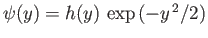varies as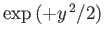as. This behavior is unacceptable, because it does not satisfy the boundary conditionas. The only way in which we can prevent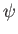from blowing up asis to demand that the power series (C.115) terminate at some finite value of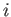. This implies, from the recursion relation (C.116), that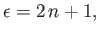(C.119)

where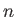is a non-negative integer. Note that the number of terms in the power series (C.115) is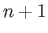. Finally, using Equation (C.109), we obtain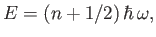(C.120)

for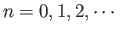.

Hence, we conclude that a particle moving in a harmonic potential has quantized energy levels that are equally spaced. The spacing between successive energy levels is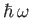, whereis the classical oscillation frequency. Furthermore, the lowest energy state (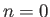) possesses the finite energy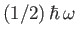. This is sometimes called zero-point energy. It is easily demonstrated that the (normalized) wavefunction of the lowest-energy state takes the form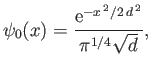(C.121)

where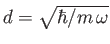.Next: Angular Momentum Up: Wave Mechanics Previous: Three-Dimensional Wave Mechanics
Richard Fitzpatrick 2016-01-25